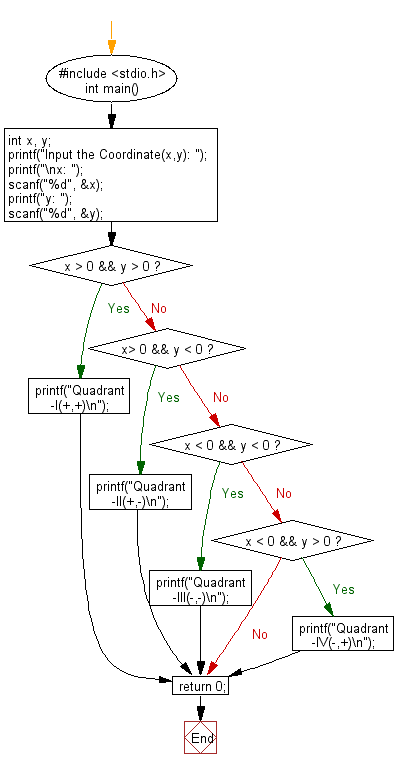﻿ C Program: Find the quadrant to which a coordinate belongs - w3resource

# C Exercises: Find the quadrant to which a coordinate belongs

## C Basic Declarations and Expressions: Exercise-37 with Solution

Note: A Cartesian coordinate system is a coordinate system that specifies each point uniquely in a plane by a pair of numerical coordinates.
These are often numbered from 1st to 4th and denoted by Roman numerals: I (where the signs of the (x,y) coordinates are I(+,+), II (−,+), III (−,−), and IV (+,−).

Sample Solution:

C Code:

``````#include <stdio.h>
int main() {
int x, y;

// Prompt for user input
printf("Input the Coordinate(x,y): ");
printf("\nx: ");
scanf("%d", &x);
printf("y: ");
scanf("%d", &y);

// Check which quadrant the point lies in
if(x > 0 && y > 0) {
}
else if(x > 0 && y < 0) {
}
else if(x < 0 && y < 0) {
}
else if(x < 0 && y > 0) {
}

return 0;
}
``````

Sample Output:

```Input the Coordinate(x,y):
x: 25
y: 15
```

Flowchart:C programming Code Editor:

What is the difficulty level of this exercise?

Test your Programming skills with w3resource's quiz.

﻿